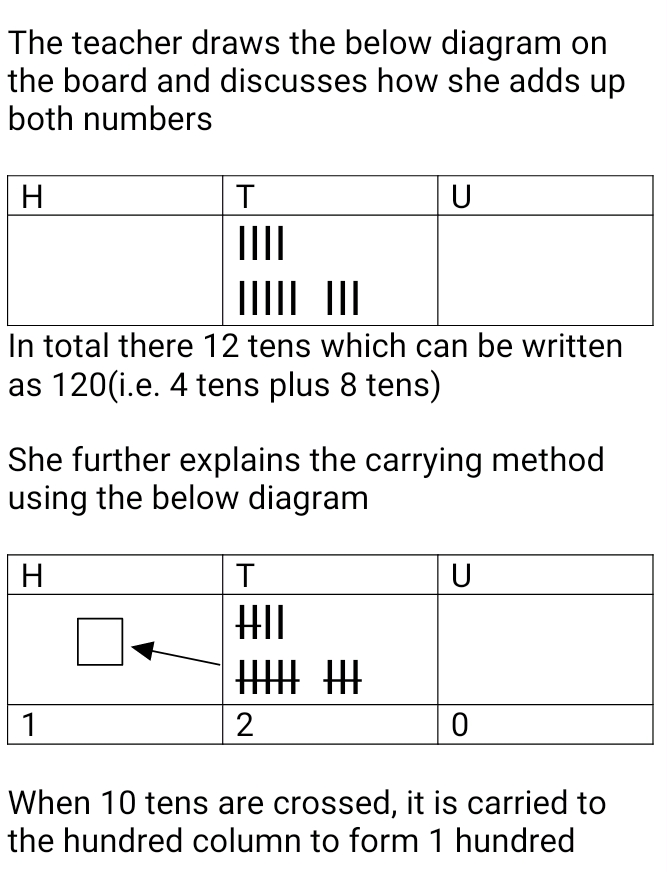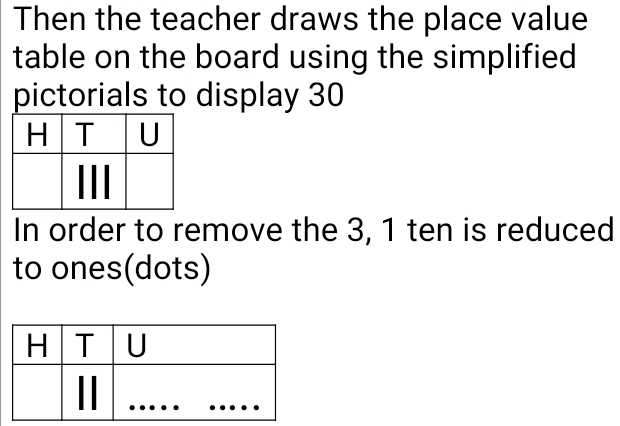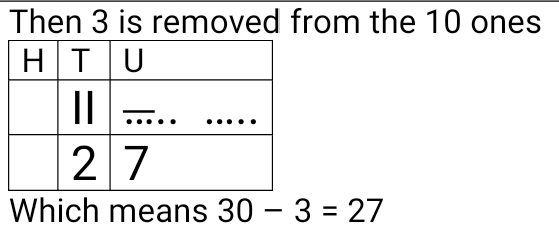# Lesson Notes By Weeks and Term - Primary 3

TERM: 1st Term

WEEK: 4

CLASS: Primary 3

AGE: 8 years

DURATION: 5 periods of 40 minutes each

DATE:

SUBJECT: Mathematics

SPECIFIC OBJECTIVES: At the end of the lesson, the pupils should be able to

1. Add and subtract up to 1000 using multiples of 10 and 100

INSTRUCTIONAL TECHNIQUES: Explanation, question and answer, demonstration, practical, assessments

INSTRUCTIONAL MATERIALS: Base 10 kit, 100 number board, scrap paper, videos from source- https://www.youtube.com/watch?v=wWaP5wk8F1A

PERIOD 1: Addition and subtraction of multiples of 10

 PRESENTATION TEACHER’S ACTIVITY PUPIL’S ACTIVITY STEP 1MENTAL MATHS The teacher begins the lesson with some mental calculationsCalculate1. 6 + ____ = 102. 3 + ____ = 103. 2 + ____ = 104. 1 + ____ = 105. 5 + ____ = 106. 5 + 6 = _____7. 9 + 5 = _____8. 4 + 8 = _____9. 13 – 6 = _____10. 16 – 7 = _____ Pupils respond and participate STEP 2CONCEPTDEVELOPMENT The teacher writes 40 + 80 = _______ on the boardWith the base 10 kits available, the pupils are asked to answer the questions individually. The learners who got the answers correctly are then asked to share the strategies they used in deriving their answers with the classCLASS ACTIVITYThe learners are asked to use the methods explained by the teacher to attempt these questions1. 50 + 90 = _____2. 140 – 90 =_____3. 200 + 300 =_____4. 600 – 400 = _____ Pupils pay attention and participate STEP 3CLASS-WORK Using the place value table, calculatea. 20 + 90 = _____b. 130 – 50 = ______c. 200 + 600 = ______d. 900 – 200 = ______e. 60 + 70 = ______ Pupils attempt their class work STEP 4HOME-WORK Using the place value tablea. 40 + 90 = ______b. 700 – 300 = ______ Pupils attempt their class work STEP 5SUMMARY The teacher summarizes by ensuring the pupils solve the problems mentally and give assistance where necessary. The teacher also ensures their solid understanding of place value She marks their class works, makes corrections where necessary and commends them positively

 PRESENTATION TEACHER’S ACTIVITY PUPIL’S ACTIVITY STEP 1MENTAL MATHS The teacher begins the lesson with some mental calculationsThe pupils practice the number bonds of 101,92,83,74,65,5 Pupils respond and participate STEP 2CONCEPTDEVELOPMENT The teacher writes 13 + 7 = _____ on the board and asks the learners to solve it mentally. They also represent the addition using counters/place value table additionWhich will give 1 ten and 10 ones which equals 20 The same process is repeated for34 + 6 = _____58 + 2 = _____ CLASS ACTIVITYThe learners work in pairs. One learner calls out any number form 11 to 20, while the other learner thinks about how many more is needed to make 20. For example if the first learner calls out 16, the second learner responds by saying 4 i.e.16 + 4 = 20Learners are asked to work out the answers mentally.Other number combinations like 12, 25 etc can also be tried Pupils pay attention and participate STEP 3CLASS-WORK 1 Fill in the missing numbers:a 15 + (    ) = 20b 13 + (    ) = 20c 17 + (    ) = 20d 19 + (    ) = 20e 16 + (    ) = 20 2 Solve the following:a 82 + 8 =b 55 + 5 =c 63 + 7 =d 46 + 4 =e 21 + 9 =f 74 + 6 =g 38 + 2 =h 57 + 3 = Pupils attempt their class work STEP 4HOME-WORK Solve the following:a 41 + 9 =b 37 + 3 =c 28 + 2 =d 65 + 5 =e 84 + 6 = The pupils writes it in their homework book STEP 5SUMMARY The teacher summarizes by ensuring the pupils solve the problems mentally and give assistance where necessary. The teacher also ensures their solid understanding of place value She marks the class work, makes corrections where necessary and commends the pupils positively

PERIOD 3: Mental maths Addition with carrying

 PRESENTATION TEACHER’S ACTIVITY PUPIL’S ACTIVITY STEP 1MENTAL MATHS The teacher begins the lesson with some mental calculationsThe pupils practice the number bonds of 201,192,183,174,165,156,147,138,129,1110,10 Pupils respond and participate STEP 2CONCEPTDEVELOPMENT The teacher writes 14 + 9 = _____ on the board. The teacher explains that there are three types of solution to the questiona. counting allb. counting onc. making-a-ten using the place value table and bondsUsing bonds14 + 9 =She asks herself what number can be added to 14 to make it 20 which is 6She then breaks 9 into 6,3So 14 + 6 = 20 and 20 + 3= 23So 14 + 9 = 23 The same process is repeated with37 + 5 =63 + 8 = CLASS ACTIVITYShe writes 14 + 20 = _____ on the board and ask the learners to use bonds to answer the above question mentallyThe same process is repeated with37 + 40 =59 + 20 = Pupils pay attention and participate STEP 3CLASS-WORK 1. Using bonds and blocks, solve these problemsa. 27 + 5 = _____b. 68 + 5 = _____c. 55 + 7 = _____d. 83 + 9 = _____e. 18 + 4 = _____ 2 Solve the problems:a 48 + 3 =b 19 + 7 =c 65 + 6 =d 75 + 8 =e 13 + 9 = 3 What do we get if we add:a 13 + 80 =b 51 + 20 = Pupils attempt their class work STEP 4HOME-WORK Using blocks and bonds, solve these problemsa. 54 + 7 =b. 29 + 5 =c. 63 + 9 =d. 42 + 9 = The pupils writes it in their homework book STEP 5SUMMARY The teacher summarizes by ensuring the pupils solve the problems mentally and give assistance where necessary. The teacher also ensures their solid understanding of place value She marks the class work, make corrections where necessary and commends the pupils positively

PERIOD 4: Mental maths subtraction

 PRESENTATION TEACHER’S ACTIVITY PUPIL’S ACTIVITY STEP 1MENTAL MATHS The teacher begins the lesson with some mental calculationsThe pupils practice the number bonds by saying numbers 10 to 19 randomly and learners answer what you need to add to make it 20 Pupils respond and participate STEP 2CONCEPTDEVELOPMENT The teacher writes 30 -3 = ____ on the board. Pupils are encouraged to solve the question mentallyThen for those who got it correctly, they are asked to share their strategies with the classThe steps are repeated with60 – 7 =80 – 9 = CLASS ACTIVITYThe teacher writes 25-9= ____ on the board.Using the make-a-ten method explains that25-9 is broken into 10+ 10 - 9 + 5 Then 10 + 10 = 2020-9 = 1111 + 5 = 16 Pupils pay attention and participate STEP 3CLASS-WORK 1 Fill in the missing numbers:a 20 is 13 andb 20 is 19 andc 20 is 12 andd 20 is 16 ande 20 is 15 and 2 Solve the following:a 20 – 7 =b 70 – 5 =c 40 – 1 =d 30 – 8 =e 60 – 6 =f 80 – 9 =g 50 – 3 =h 90 – 2 = Pupils attempt their class work STEP 4HOME-WORK Using blocks and bonds, solve these problems1 Solve the following:a 30 – 1 =b 70 – 8 =c 20 – 5 =d 90 – 3 =e 60 – 4 = The pupils writes it in their homework book STEP 5SUMMARY The teacher summarizes by ensuring the pupils solve the problems mentally and give assistance where necessary. The teacher also ensures their solid understanding of place value She marks the class work, make corrections where necessary and commends the pupils positively

PERIOD 5: Weekly Test/consolidations

TEACHER’S ACTIVITY: The teacher revises all the concepts treated from period 1-4 and gives the pupils follow through exercises, quiz and tests . She marks the exercises, makes corrections and commends the pupils positively.

PUPIL’S ACTIVITY: The pupils work on the worksheets and exercises given by the teacher individually

ASSESSMENT

1 Solve the problems:

a 57 + 5 =

b 39 + 3 =

c 65 + 8 =

d 46 + 7 =

e 57 + 4 =

f 79 + 4 =

g 32 + 9 =

h 83 + 8 =

2 Fill in the missing numbers:

a 55 + ____ = 60

b 37 + ____ = 40

c 41 + ____ = 50

3 Solve the following:

a 53 + ____ = 60

b 42 + 8 =

c 27 + ____ = 30

d 88 + 2 =

4 Fill in the missing numbers:

a 20 is 14 and

b 30 is 29 and

c 40 is 32 and

5 Solve the following:

a 40 – 6 =

b 80 – 5 =

c 20 – 9 =

d 60 – 7 =